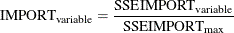Variable Importance

Variable importance is based on how the variables are used in the pruned tree. Four metrics are used: count, surrogate count, SSE, and relative importance. The count-based variable importance simply counts the number of times in the entire tree that a given variable is used in a split. Similarly, the surrogate count counts the number of times a variable is used in a surrogate splitting rule. The SSE and relative importance are calculated from the training set. They are also calculated again from the validation set if one exists.

The SSE-based variable importance is based on the nodes in which the variable is used in a split. For each variable, the change of the SSE that results from the split is found. The change is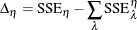where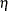denotes the node.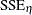is then the SSE if the node is treated as a leaf, andis the SSE of the node after it has been split. If the change in SSE is negative (which is possible when you use the validation set), then the change is set to 0.

If surrogate rules are present, they are also credited with a portion of the reduction in SSE. The credit is related to the agreement; that is, the fraction of nonmissing observations in which the primary and surrogate splitting rules send to the same children is expressed by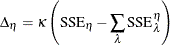where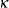is the agreement,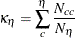where c is a child of node,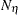is the number of nonmissing observations, and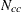is the number of observations that were assigned to c by both the primary rule and the surrogate rule.

The SSE-based importance is then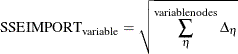The relative importance metric is based on the SSE of each variable. The maximum SSE variable importance is found. Then all the variables are assigned a relative importance, which is simply